# For beam given below Draw the shear- and moment diagrams. Label all important values of shear...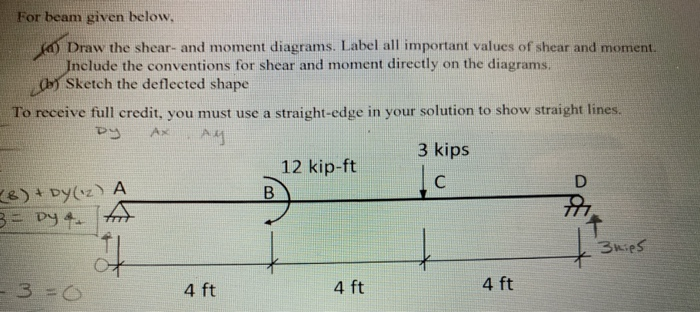For beam given below Draw the shear- and moment diagrams. Label all important values of shear and moment. nclude the conventions for shear and moment directly on the diagrams Sketch the deflected shape To reeiv leredr you mus ue astraighto sbwg ns 3 kips 12 kip-ft 4 ft 4 ft 4 ft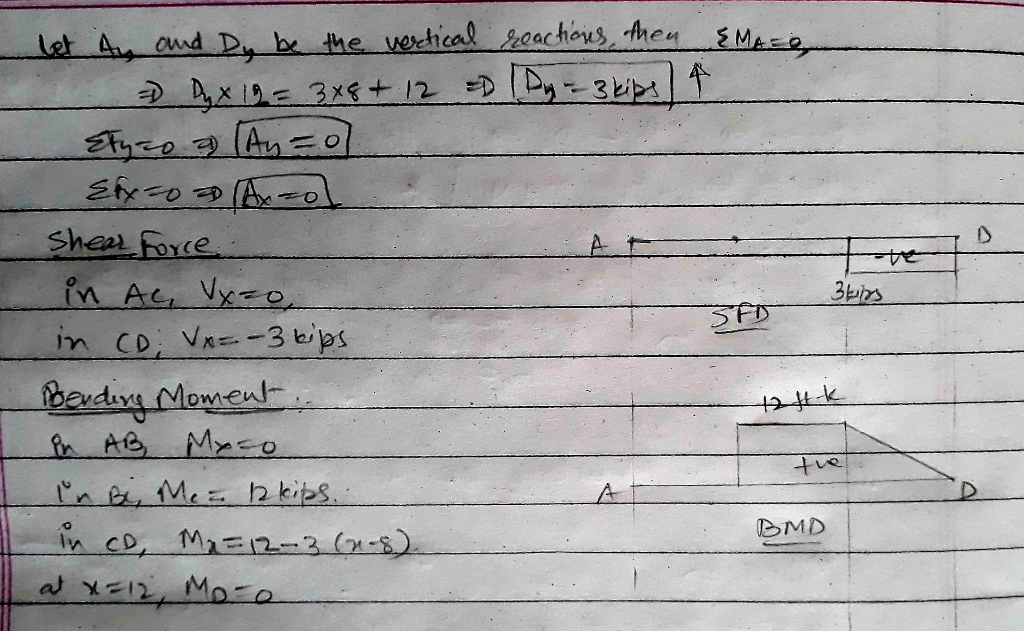#### Earn Coin

Coins can be redeemed for fabulous gifts.

Similar Homework Help Questions
• ### b. Draw shear diagram (identify all important shear values, slopes, and convention on the diagram) c....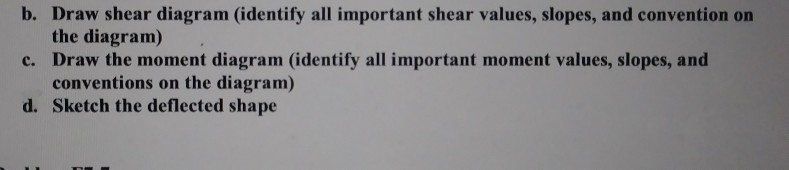b. Draw shear diagram (identify all important shear values, slopes, and convention on the diagram) c. Draw the moment diagram (identify all important moment values, slopes, and conventions on the diagram) d. Sketch the deflected shape 4 KN 12 kNm A B с 3 m 3 m Prob. F7-12

• ### 1-(25%) Draw shear and moment diagrams for the beam shown in Figure P-1 . Draw a sketch of the deflected shape. The spans are 30-ft long each (total of 90-ft), the concentrated loads are eac...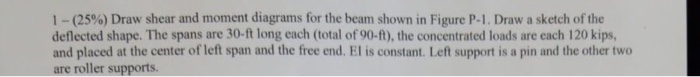1-(25%) Draw shear and moment diagrams for the beam shown in Figure P-1 . Draw a sketch of the deflected shape. The spans are 30-ft long each (total of 90-ft), the concentrated loads are each 120 kips, and placed at the center of left span and the free end. El is constant. Left support is a pin and the other two are roller supports. 120 kips 120 kips Figure P-1 1-(25%) Draw shear and moment diagrams for the beam shown...

• ### 2) For the beam shown below, plot the shear force and bending moment diagrams using the...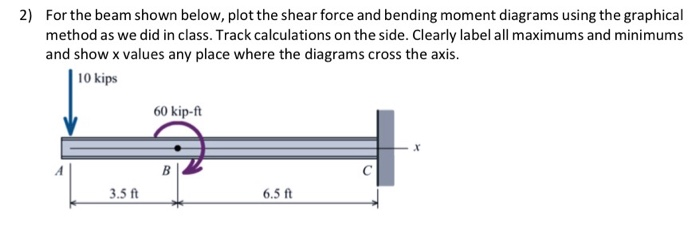2) For the beam shown below, plot the shear force and bending moment diagrams using the graphical method as we did in class. Track calculations on the side. Clearly label all maximums and minimums and show x values any place where the diagrams cross the axis. | 10 kips 60 kip-ft 6.5 ft

• ### b. Draw shear diagram (identify all important shear values, slopes, and convention on the diagram) c....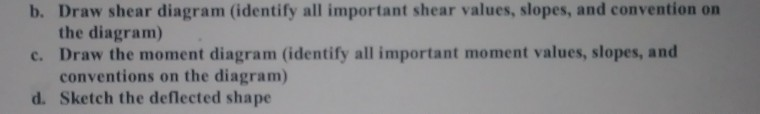b. Draw shear diagram (identify all important shear values, slopes, and convention on the diagram) c. Draw the moment diagram (identify all important moment values, slopes, and conventions on the diagram) d. Sketch the deflected shape 6 kN A 3 m Prob. F7-7

• ### pls do all parts a b and c b. Draw shear diagram (identify all important shear...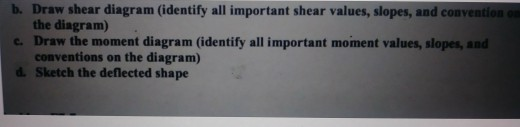pls do all parts a b and c b. Draw shear diagram (identify all important shear values, slopes, and convention the diagram) c. Draw the moment diagram (identify all important moment values, slopes, and conventions on the diagram) d. Sketch the deflected shape 4 kN 12 kNm A B IC 3 m 3 m Prob. F7-12

• ### b. Draw shear diagram (identify all important shear values, slopes, and convention on the diagram) c....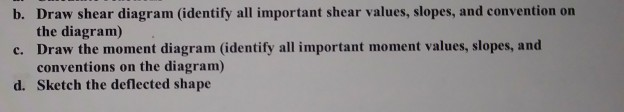b. Draw shear diagram (identify all important shear values, slopes, and convention on the diagram) c. Draw the moment diagram (identify all important moment values, slopes, and conventions on the diagram) d. Sketch the deflected shape 6 KN 8 kN/m A 1.5 m 1.5 m Prob. F7-14

• ### Please find all reactions with Moment Distribution Method & draw shear/moment diagrams. Please show all work. Thank you in advance! Problem #2: For the indeterminate beam shown below: a) Determin...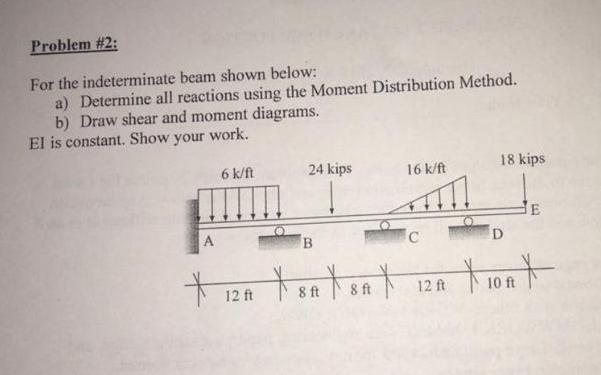Please find all reactions with Moment Distribution Method & draw shear/moment diagrams. Please show all work. Thank you in advance! Problem #2: For the indeterminate beam shown below: a) Determine all reactions using the Moment Distribution Method. b) Draw shear and moment diagrams. El is constant. Show your work. 18 kips 24 kips 6 k/ft 16 k/ft 10ft 12 ft 12ft Problem #2: For the indeterminate beam shown below: a) Determine all reactions using the Moment Distribution Method. b) Draw...

• ### QUESTION 4 4. Bending Moment in a Beam Draw the shear and bending moment diagrams for...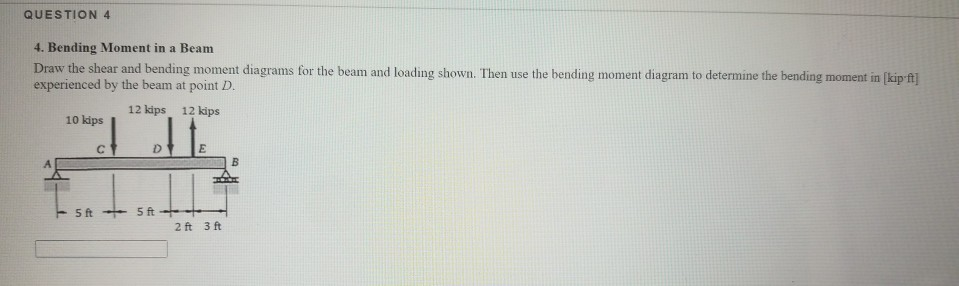QUESTION 4 4. Bending Moment in a Beam Draw the shear and bending moment diagrams for the beam and loading shown. Then use the bending moment diagram to determine the bending moment in ſkip-ft] experienced by the beam at point D. 12 kips 12 kips 10 kips the С - 5 ft 5ft --- 2 ft 3 ft

• ### 10 For beam AB, (a) draw the shear and bending-moment diagrams, the location and magn absolute...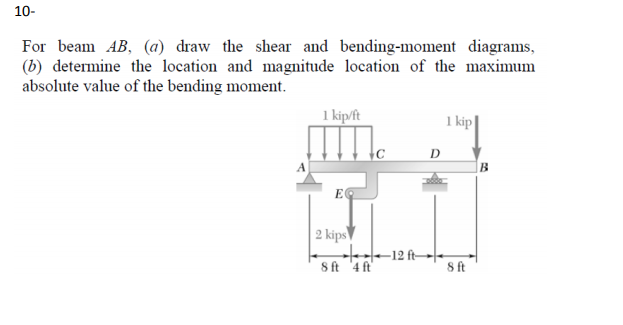10 For beam AB, (a) draw the shear and bending-moment diagrams, the location and magn absolute value of the bending moment. l kip/ft 1 kip 2 kips Sft 4ft s ft

• ### A) Draw the shear and bending-moment diagrams for the beam and loading shown. B)Determine the magnitude...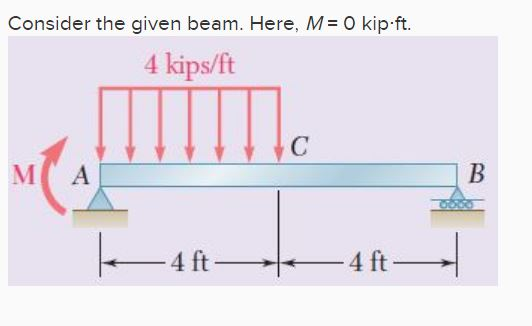A) Draw the shear and bending-moment diagrams for the beam and loading shown. B)Determine the magnitude and location of the maximum absolute value of the bending moment. (Round the final answer to the nearest whole number.) The magnitude of the bending moment, |M|max is _______ kip·ft and it is located _______ ft from A. Consider the given beam. Here, M O kip ft. 4 kips/ft MC A 4ft4 ft-